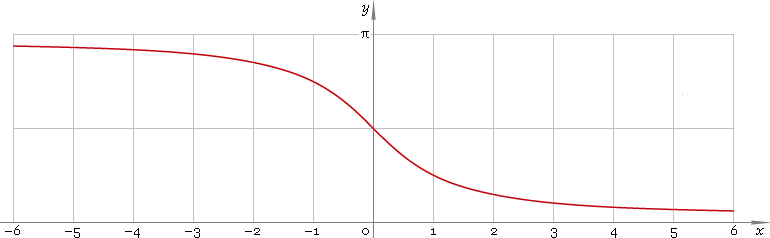The Art of Mathematics

# arccot or arcctg — trigonometric arc cotangent function

## 1. Definition

Arc cotangent is inverse of the cotangent function.

arccotxcotinvx

## 2. Graph

Arc cotangent is monotone function defined everywhere on real axis. Its graph is depicted below — fig. 1.Fig. 1. Graph of the arc cotangent function y = arccotx.

Function codomain is limited to the range (0, π).

## 3. Identities

Complementary angle:

arctanx + arccotx = π/2

and as consequence:

arccot tan φ = π/2 − φ

Negative argument:

arccot(−x) = π − arccotx

Reciprocal argument:

arccot(1/x) = arctanx for x > 0,
arccot(1/x) = arctanx + π for x < 0

Sum and difference:

arccotx + arccoty = arccot[(xy − 1) /(x + y)]
arccotx − arccoty = arccot[(xy + 1) /(yx)]

Some argument values:

Argument xValue arccotx
0π/2
2 − √35π/12
√(1 − 2 /√5)2π/5
√2 − 13π/8
√3 /3π/3
√(5 − 2 √5)3π/10
1π/4
√(1 + 2 /√5)π/5
√3π/6
√2 + 1π/8
√(5 + 2 √5)π/10
2 + √3π/12
Table 1. Arc cotangent for some argument values.

## 4. Derivative and indefinite integral

Arc cotangent derivative:

arccot′x = −1 /(1 + x2)

Indefinite integral of the arc cotangent:

∫ arccotx dx = x arccotx + ln(1 + x2) /2 + C

where C is an arbitrary constant.

## 5. How to use

To calculate arc cotangent of the number:

``arccot(−1);``

To get arc cotangent of the complex number:

``arccot(−1+i);``

To get arc cotangent of the current result:

``arccot(rslt);``

To get arc cotangent of the number z in calculator memory:

``arccot(mem[z]);``

## 6. Support

Trigonometric arc cotangent of the real argument is supported in free version of the Librow calculator.

Trigonometric arc cotangent of the complex argument is supported in professional version of the Librow calculator.